# Full infer Pipeline Examples

#### Introduction

This vignette is intended to provide a set of examples that nearly exhaustively demonstrate the functionalities provided by `infer`. Commentary on these examples is limited—for more discussion of the intuition behind the package, see the “Getting to Know infer” vignette, accessible by calling `vignette("infer")`.

Throughout this vignette, we’ll make use of the `gss` dataset supplied by `infer`, which contains a sample of data from the General Social Survey. See `?gss` for more information on the variables included and their source. Note that this data (and our examples on it) are for demonstration purposes only, and will not necessarily provide accurate estimates unless weighted properly. For these examples, let’s suppose that this dataset is a representative sample of a population we want to learn about: American adults. The data looks like this:

``````# load in the dataset
data(gss)

# take a look at its structure
dplyr::glimpse(gss)``````
``````## Rows: 500
## Columns: 11
## \$ year    <dbl> 2014, 1994, 1998, 1996, 1994, 1996, 1990, 2016, 2000, 1998, 20…
## \$ age     <dbl> 36, 34, 24, 42, 31, 32, 48, 36, 30, 33, 21, 30, 38, 49, 25, 56…
## \$ sex     <fct> male, female, male, male, male, female, female, female, female…
## \$ college <fct> degree, no degree, degree, no degree, degree, no degree, no de…
## \$ partyid <fct> ind, rep, ind, ind, rep, rep, dem, ind, rep, dem, dem, ind, de…
## \$ hompop  <dbl> 3, 4, 1, 4, 2, 4, 2, 1, 5, 2, 4, 3, 4, 4, 2, 2, 3, 2, 1, 2, 5,…
## \$ hours   <dbl> 50, 31, 40, 40, 40, 53, 32, 20, 40, 40, 23, 52, 38, 72, 48, 40…
## \$ income  <ord> \$25000 or more, \$20000 - 24999, \$25000 or more, \$25000 or more…
## \$ class   <fct> middle class, working class, working class, working class, mid…
## \$ finrela <fct> below average, below average, below average, above average, ab…
## \$ weight  <dbl> 0.8960, 1.0825, 0.5501, 1.0864, 1.0825, 1.0864, 1.0627, 0.4785…``````

## Hypothesis tests

### One numerical variable (mean)

Calculating the observed statistic,

``````x_bar <- gss %>%
specify(response = hours) %>%
calculate(stat = "mean")``````

Alternatively, using the `observe()` wrapper to calculate the observed statistic,

``````x_bar <- gss %>%
observe(response = hours, stat = "mean")``````

Then, generating the null distribution,

``````null_dist <- gss %>%
specify(response = hours) %>%
hypothesize(null = "point", mu = 40) %>%
generate(reps = 1000) %>%
calculate(stat = "mean")``````

Visualizing the observed statistic alongside the null distribution,

``````visualize(null_dist) +
shade_p_value(obs_stat = x_bar, direction = "two-sided")``````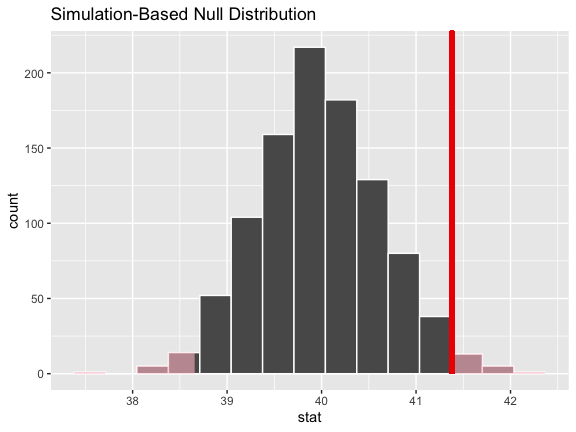Calculating the p-value from the null distribution and observed statistic,

``````null_dist %>%
get_p_value(obs_stat = x_bar, direction = "two-sided")``````
p_value
0.038

### One numerical variable (standardized mean \(t\))

Calculating the observed statistic,

``````t_bar <- gss %>%
specify(response = hours) %>%
hypothesize(null = "point", mu = 40) %>%
calculate(stat = "t")``````

Alternatively, using the `observe()` wrapper to calculate the observed statistic,

``````t_bar <- gss %>%
observe(response = hours, null = "point", mu = 40, stat = "t")``````

Then, generating the null distribution,

``````null_dist <- gss %>%
specify(response = hours) %>%
hypothesize(null = "point", mu = 40) %>%
generate(reps = 1000) %>%
calculate(stat = "t")``````

Alternatively, finding the null distribution using theoretical methods using the `assume()` verb,

``````null_dist_theory <- gss %>%
specify(response = hours)  %>%
assume("t")``````

Visualizing the observed statistic alongside the null distribution,

``````visualize(null_dist) +
shade_p_value(obs_stat = t_bar, direction = "two-sided")``````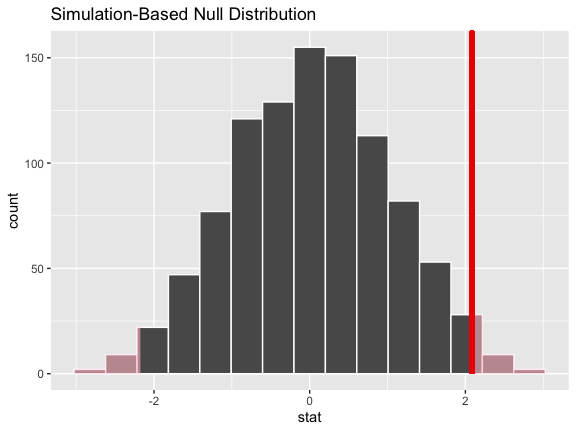Alternatively, visualizing the observed statistic using the theory-based null distribution,

``````visualize(null_dist_theory) +
shade_p_value(obs_stat = t_bar, direction = "two-sided")``````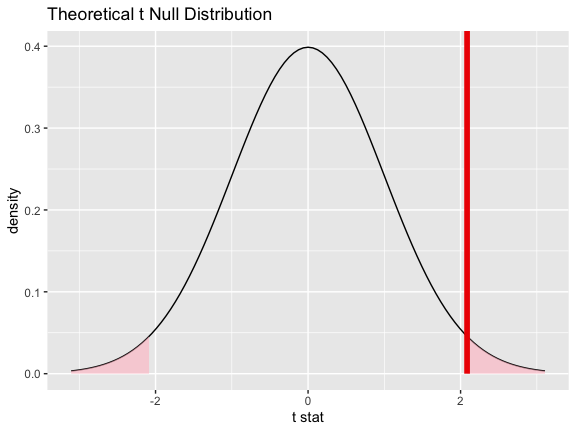Alternatively, visualizing the observed statistic using both of the null distributions,

``````visualize(null_dist, method = "both") +
shade_p_value(obs_stat = t_bar, direction = "two-sided")``````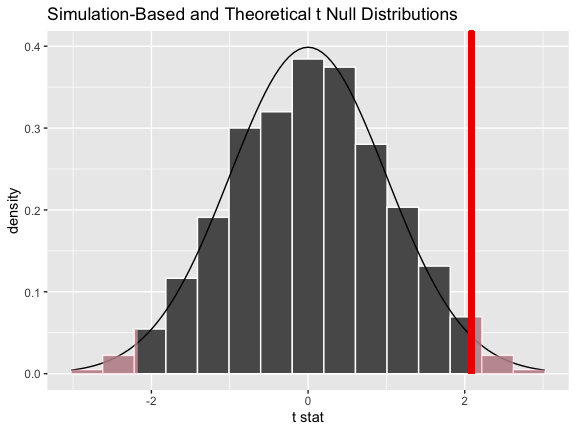Note that the above code makes use of the randomization-based null distribution.

Calculating the p-value from the null distribution and observed statistic,

``````null_dist %>%
get_p_value(obs_stat = t_bar, direction = "two-sided")``````
p_value
0.028

Alternatively, using the `t_test` wrapper:

``````gss %>%
t_test(response = hours, mu = 40)``````
statistic t_df p_value alternative estimate lower_ci upper_ci
2.085 499 0.0376 two.sided 41.38 40.08 42.68

`infer` does not support testing on one numerical variable via the `z` distribution.

### One numerical variable (median)

Calculating the observed statistic,

``````x_tilde <- gss %>%
specify(response = age) %>%
calculate(stat = "median")``````

Alternatively, using the `observe()` wrapper to calculate the observed statistic,

``````x_tilde <- gss %>%
observe(response = age, stat = "median")``````

Then, generating the null distribution,

``````null_dist <- gss %>%
specify(response = age) %>%
hypothesize(null = "point", med = 40) %>%
generate(reps = 1000) %>%
calculate(stat = "median")``````

Visualizing the observed statistic alongside the null distribution,

``````visualize(null_dist) +
shade_p_value(obs_stat = x_tilde, direction = "two-sided")``````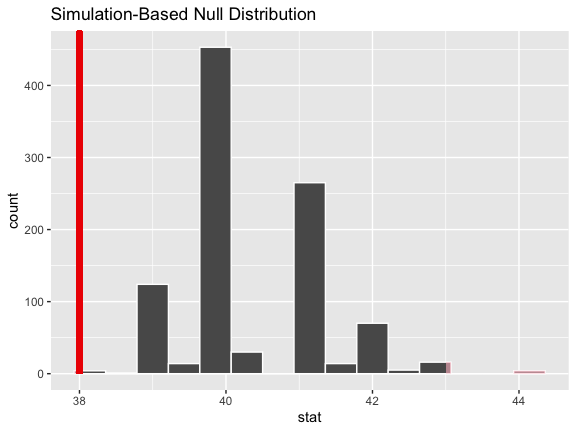Calculating the p-value from the null distribution and observed statistic,

``````null_dist %>%
get_p_value(obs_stat = x_tilde, direction = "two-sided")``````
p_value
0.008

### One numerical variable (paired)

The example under this header is compatible with `stat`s `"mean"`, `"median"`, `"sum"`, and `"sd"`.

Suppose that each of these survey respondents had provided the number of `hours` worked per week when surveyed 5 years prior, encoded as `hours_previous`.

``````set.seed(1)

gss_paired <- gss %>%
mutate(
hours_previous = hours + 5 - rpois(nrow(.), 4.8),
diff = hours - hours_previous
)

gss_paired %>%
select(hours, hours_previous, diff)``````
hours hours_previous diff
50 52 -2
31 32 -1
40 40 0
40 37 3
40 42 -2
53 50 3
32 28 4
20 19 1
40 40 0
40 43 -3
23 25 -2
52 54 -2
38 37 1
72 73 -1
48 47 1
40 40 0
40 39 1
28 22 6
30 31 -1
40 39 1
40 37 3
20 22 -2
40 39 1
3 6 -3
55 57 -2
60 61 -1
40 44 -4
71 72 -1
50 48 2
32 33 -1
40 40 0
50 50 0
50 50 0
72 74 -2
42 40 2
40 39 1
40 38 2
40 43 -3
42 41 1
56 57 -1
30 28 2
20 20 0
15 14 1
40 40 0
56 56 0
40 39 1
72 76 -4
40 40 0
30 29 1
40 39 1
44 44 0
40 38 2
36 37 -1
40 42 -2
89 92 -3
50 53 -3
40 41 -1
40 40 0
50 49 1
40 41 -1
65 62 3
45 47 -2
40 41 -1
42 43 -1
40 40 0
40 42 -2
36 36 0
50 49 1
40 43 -3
30 28 2
20 21 -1
35 33 2
40 41 -1
50 51 -1
50 50 0
37 34 3
35 33 2
16 17 -1
60 59 1
40 36 4
55 56 -1
56 55 1
72 73 -1
40 41 -1
52 51 1
40 42 -2
6 5 1
78 81 -3
13 15 -2
44 46 -2
20 22 -2
8 11 -3
40 40 0
40 38 2
60 59 1
40 38 2
15 16 -1
60 61 -1
40 38 2
14 14 0
40 39 1
20 21 -1
40 42 -2
50 44 6
24 24 0
48 50 -2
40 43 -3
48 48 0
48 45 3
50 50 0
40 35 5
40 39 1
48 49 -1
44 45 -1
10 12 -2
40 44 -4
35 34 1
40 43 -3
12 13 -1
40 40 0
40 34 6
60 60 0
30 30 0
35 37 -2
56 55 1
45 46 -1
46 46 0
45 47 -2
40 42 -2
40 40 0
89 89 0
40 43 -3
59 63 -4
43 43 0
40 37 3
44 44 0
20 20 0
20 20 0
45 40 5
12 12 0
40 39 1
37 37 0
40 42 -2
15 17 -2
30 29 1
38 39 -1
41 43 -2
50 49 1
30 33 -3
40 38 2
40 40 0
40 40 0
45 46 -1
40 41 -1
53 53 0
32 34 -2
40 40 0
56 59 -3
40 42 -2
40 42 -2
40 42 -2
40 37 3
40 41 -1
10 9 1
45 43 2
34 35 -1
45 48 -3
65 66 -1
48 47 1
40 41 -1
27 27 0
40 38 2
50 48 2
40 41 -1
40 41 -1
50 47 3
50 50 0
15 14 1
40 40 0
20 17 3
43 45 -2
20 22 -2
38 36 2
40 40 0
40 38 2
40 42 -2
40 39 1
56 55 1
43 40 3
53 53 0
32 31 1
25 26 -1
40 43 -3
40 37 3
40 42 -2
40 40 0
45 48 -3
32 30 2
32 33 -1
38 37 1
60 62 -2
27 29 -2
43 43 0
89 91 -2
48 50 -2
40 40 0
40 40 0
20 23 -3
20 22 -2
30 29 1
60 56 4
56 59 -3
40 39 1
40 36 4
45 43 2
72 73 -1
40 40 0
51 47 4
40 36 4
60 61 -1
24 26 -2
40 42 -2
40 41 -1
80 80 0
24 21 3
40 40 0
30 32 -2
52 56 -4
50 51 -1
22 20 2
40 41 -1
35 38 -3
37 38 -1
50 50 0
47 48 -1
30 29 1
40 39 1
25 25 0
35 36 -1
27 28 -1
40 41 -1
30 30 0
36 33 3
40 42 -2
48 49 -1
40 42 -2
40 41 -1
30 33 -3
40 41 -1
63 60 3
40 39 1
30 27 3
40 41 -1
89 89 0
55 55 0
6 9 -3
40 42 -2
50 50 0
64 65 -1
10 7 3
45 45 0
40 41 -1
40 42 -2
40 39 1
15 14 1
45 47 -2
75 78 -3
38 37 1
75 75 0
8 10 -2
40 43 -3
12 15 -3
55 56 -1
10 12 -2
40 41 -1
55 57 -2
40 42 -2
40 42 -2
43 43 0
35 34 1
18 22 -4
48 48 0
60 58 2
60 61 -1
40 43 -3
45 48 -3
40 41 -1
44 46 -2
40 43 -3
40 42 -2
50 52 -2
56 59 -3
50 45 5
55 56 -1
20 20 0
40 39 1
45 48 -3
35 38 -3
40 43 -3
40 37 3
50 49 1
40 43 -3
48 48 0
50 51 -1
65 66 -1
46 40 6
40 42 -2
16 14 2
35 38 -3
40 41 -1
75 78 -3
50 52 -2
40 38 2
22 21 1
50 52 -2
40 40 0
40 43 -3
89 90 -1
40 36 4
43 43 0
45 44 1
40 41 -1
40 41 -1
48 41 7
40 38 2
60 56 4
45 43 2
40 39 1
40 42 -2
5 4 1
60 55 5
70 72 -2
50 51 -1
40 38 2
60 63 -3
37 38 -1
5 6 -1
40 42 -2
24 24 0
40 36 4
80 75 5
60 62 -2
36 36 0
50 51 -1
25 24 1
40 42 -2
30 31 -1
38 40 -2
50 51 -1
40 39 1
57 57 0
40 43 -3
40 39 1
50 51 -1
36 31 5
80 82 -2
55 53 2
40 43 -3
64 62 2
45 46 -1
40 43 -3
40 41 -1
25 23 2
89 91 -2
40 40 0
40 38 2
40 43 -3
15 14 1
23 22 1
48 49 -1
55 56 -1
27 27 0
52 49 3
40 42 -2
40 42 -2
41 42 -1
40 41 -1
38 36 2
44 46 -2
50 50 0
40 41 -1
56 56 0
40 39 1
40 35 5
42 44 -2
40 42 -2
70 68 2
48 46 2
30 33 -3
50 46 4
16 18 -2
42 44 -2
40 37 3
50 50 0
60 62 -2
35 36 -1
40 39 1
40 41 -1
40 41 -1
40 42 -2
19 18 1
40 42 -2
40 36 4
40 37 3
40 37 3
30 29 1
70 71 -1
30 29 1
40 44 -4
20 17 3
70 64 6
50 51 -1
56 55 1
40 38 2
39 38 1
6 7 -1
25 25 0
30 31 -1
40 39 1
30 28 2
20 21 -1
60 60 0
45 44 1
48 50 -2
60 61 -1
40 41 -1
55 58 -3
38 35 3
57 55 2
35 33 2
27 24 3
50 53 -3
15 16 -1
40 40 0
45 48 -3
40 38 2
28 27 1
60 63 -3
40 40 0
50 47 3
4 8 -4
40 42 -2
44 44 0
40 40 0
35 37 -2
50 51 -1
43 44 -1
70 67 3
40 43 -3
40 43 -3
20 16 4
40 41 -1
40 41 -1
20 22 -2
37 40 -3
88 87 1
44 44 0
30 25 5
40 40 0
24 27 -3
30 32 -2
80 81 -1
40 45 -5
40 41 -1
60 62 -2
80 77 3
40 39 1
15 17 -2
43 44 -1
50 49 1
40 41 -1
40 36 4
53 52 1
22 23 -1
30 33 -3
40 42 -2
12 10 2
86 87 -1
45 45 0
25 24 1
30 29 1
55 57 -2
50 48 2
40 36 4
20 20 0
25 25 0
40 42 -2
40 45 -5
25 23 2
40 43 -3
40 44 -4
30 27 3
40 42 -2
40 42 -2
40 41 -1
21 22 -1
75 76 -1
40 40 0
40 38 2
40 43 -3

We’d like to test the null hypothesis that the `"mean"` hours worked per week did not change between the sampled time and five years prior.

infer supports paired hypothesis testing via the `null = "paired independence"` argument to `hypothesize()`.

Calculating the observed statistic,

``````x_tilde <- gss_paired %>%
specify(response = diff) %>%
calculate(stat = "mean")``````

Alternatively, using the `observe()` wrapper to calculate the observed statistic,

``````x_tilde <- gss_paired %>%
observe(response = diff, stat = "mean")``````

Then, generating the null distribution,

``````null_dist <- gss_paired %>%
specify(response = diff) %>%
hypothesize(null = "paired independence") %>%
generate(reps = 1000, type = "permute") %>%
calculate(stat = "mean")``````

Note that the `diff` column itself is not permuted, but rather the signs of the values in the column.

Visualizing the observed statistic alongside the null distribution,

``````visualize(null_dist) +
shade_p_value(obs_stat = x_tilde, direction = "two-sided")``````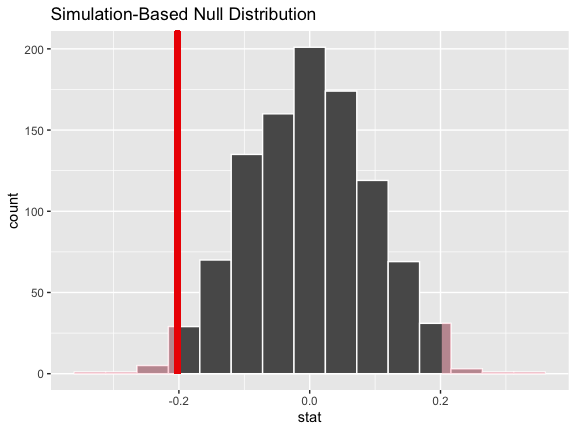Calculating the p-value from the null distribution and observed statistic,

``````null_dist %>%
get_p_value(obs_stat = x_tilde, direction = "two-sided")``````
p_value
0.028

### One categorical (one proportion)

Calculating the observed statistic,

``````p_hat <- gss %>%
specify(response = sex, success = "female") %>%
calculate(stat = "prop")``````

Alternatively, using the `observe()` wrapper to calculate the observed statistic,

``````p_hat <- gss %>%
observe(response = sex, success = "female", stat = "prop")``````

Then, generating the null distribution,

``````null_dist <- gss %>%
specify(response = sex, success = "female") %>%
hypothesize(null = "point", p = .5) %>%
generate(reps = 1000) %>%
calculate(stat = "prop")``````

Visualizing the observed statistic alongside the null distribution,

``````visualize(null_dist) +
shade_p_value(obs_stat = p_hat, direction = "two-sided")``````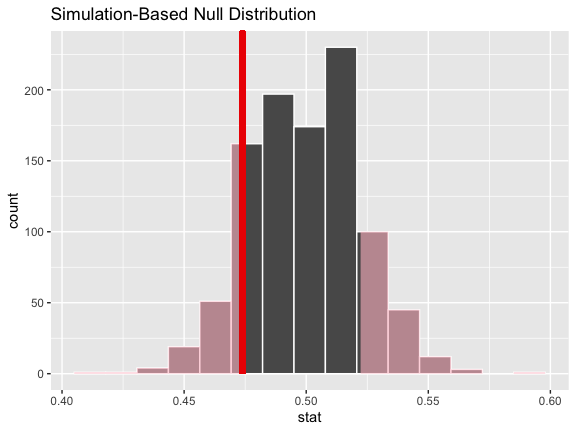Calculating the p-value from the null distribution and observed statistic,

``````null_dist %>%
get_p_value(obs_stat = p_hat, direction = "two-sided")``````
p_value
0.276

Note that logical variables will be coerced to factors:

``````null_dist <- gss %>%
dplyr::mutate(is_female = (sex == "female")) %>%
specify(response = is_female, success = "TRUE") %>%
hypothesize(null = "point", p = .5) %>%
generate(reps = 1000) %>%
calculate(stat = "prop")``````

### One categorical variable (standardized proportion \(z\))

Calculating the observed statistic,

``````p_hat <- gss %>%
specify(response = sex, success = "female") %>%
hypothesize(null = "point", p = .5) %>%
calculate(stat = "z")``````

Alternatively, using the `observe()` wrapper to calculate the observed statistic,

``````p_hat <- gss %>%
observe(response = sex, success = "female", null = "point", p = .5, stat = "z")``````

Then, generating the null distribution,

``````null_dist <- gss %>%
specify(response = sex, success = "female") %>%
hypothesize(null = "point", p = .5) %>%
generate(reps = 1000, type = "draw") %>%
calculate(stat = "z")``````

Visualizing the observed statistic alongside the null distribution,

``````visualize(null_dist) +
shade_p_value(obs_stat = p_hat, direction = "two-sided")``````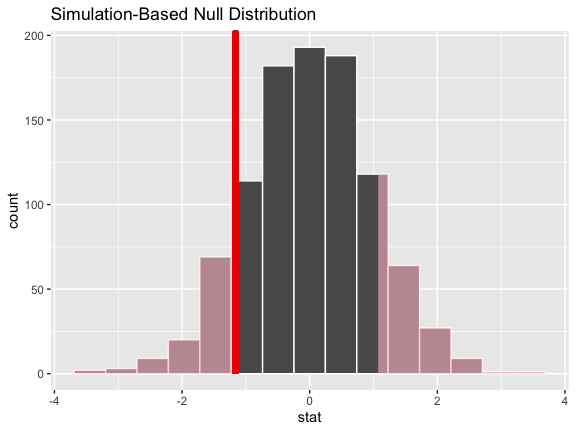Calculating the p-value from the null distribution and observed statistic,

``````null_dist %>%
get_p_value(obs_stat = p_hat, direction = "two-sided")``````
p_value
0.252

The package also supplies a wrapper around `prop.test` for tests of a single proportion on tidy data.

``````prop_test(gss,
college ~ NULL,
p = .2)``````
statistic chisq_df p_value alternative
635.6 1 0 two.sided

`infer` does not support testing two means via the `z` distribution.

### Two categorical (2 level) variables

The `infer` package provides several statistics to work with data of this type. One of them is the statistic for difference in proportions.

Calculating the observed statistic,

``````d_hat <- gss %>%
specify(college ~ sex, success = "no degree") %>%
calculate(stat = "diff in props", order = c("female", "male"))``````

Alternatively, using the `observe()` wrapper to calculate the observed statistic,

``````d_hat <- gss %>%
observe(college ~ sex, success = "no degree",
stat = "diff in props", order = c("female", "male"))``````

Then, generating the null distribution,

``````null_dist <- gss %>%
specify(college ~ sex, success = "no degree") %>%
hypothesize(null = "independence") %>%
generate(reps = 1000) %>%
calculate(stat = "diff in props", order = c("female", "male"))``````

Visualizing the observed statistic alongside the null distribution,

``````visualize(null_dist) +
shade_p_value(obs_stat = d_hat, direction = "two-sided")``````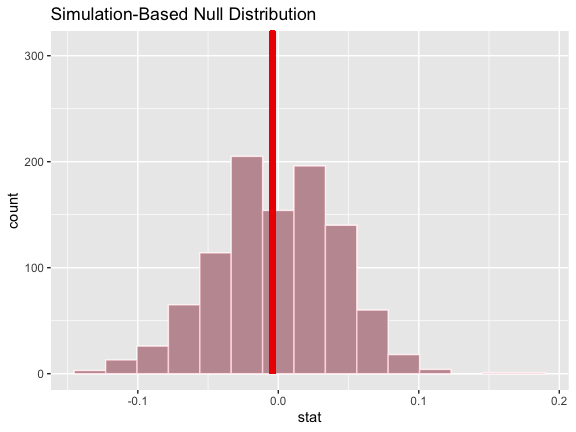Calculating the p-value from the null distribution and observed statistic,

``````null_dist %>%
get_p_value(obs_stat = d_hat, direction = "two-sided")``````
p_value
1

`infer` also provides functionality to calculate ratios of proportions. The workflow looks similar to that for `diff in props`.

Calculating the observed statistic,

``````r_hat <- gss %>%
specify(college ~ sex, success = "no degree") %>%
calculate(stat = "ratio of props", order = c("female", "male"))``````

Alternatively, using the `observe()` wrapper to calculate the observed statistic,

``````r_hat <- gss %>%
observe(college ~ sex, success = "no degree",
stat = "ratio of props", order = c("female", "male"))``````

Then, generating the null distribution,

``````null_dist <- gss %>%
specify(college ~ sex, success = "no degree") %>%
hypothesize(null = "independence") %>%
generate(reps = 1000) %>%
calculate(stat = "ratio of props", order = c("female", "male"))``````

Visualizing the observed statistic alongside the null distribution,

``````visualize(null_dist) +
shade_p_value(obs_stat = r_hat, direction = "two-sided")``````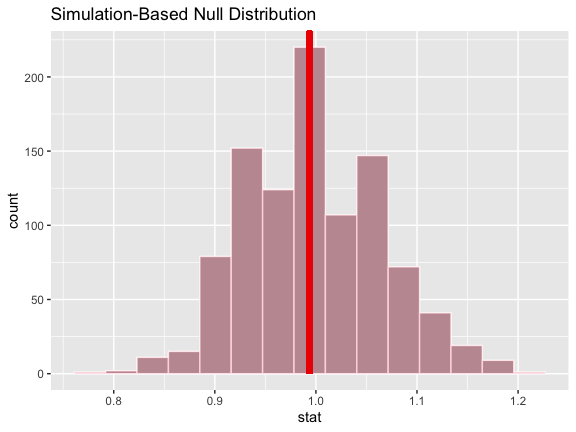Calculating the p-value from the null distribution and observed statistic,

``````null_dist %>%
get_p_value(obs_stat = r_hat, direction = "two-sided")``````
p_value
1

In addition, the package provides functionality to calculate odds ratios. The workflow also looks similar to that for `diff in props`.

Calculating the observed statistic,

``````or_hat <- gss %>%
specify(college ~ sex, success = "no degree") %>%
calculate(stat = "odds ratio", order = c("female", "male"))``````

Then, generating the null distribution,

``````null_dist <- gss %>%
specify(college ~ sex, success = "no degree") %>%
hypothesize(null = "independence") %>%
generate(reps = 1000) %>%
calculate(stat = "odds ratio", order = c("female", "male"))``````

Visualizing the observed statistic alongside the null distribution,

``````visualize(null_dist) +
shade_p_value(obs_stat = or_hat, direction = "two-sided")``````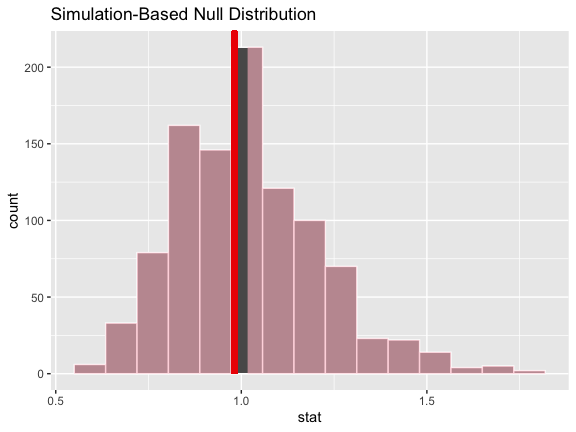Calculating the p-value from the null distribution and observed statistic,

``````null_dist %>%
get_p_value(obs_stat = or_hat, direction = "two-sided")``````
p_value
0.984

### Two categorical (2 level) variables (z)

Finding the standardized observed statistic,

``````z_hat <- gss %>%
specify(college ~ sex, success = "no degree") %>%
hypothesize(null = "independence") %>%
calculate(stat = "z", order = c("female", "male"))``````

Alternatively, using the `observe()` wrapper to calculate the observed statistic,

``````z_hat <- gss %>%
observe(college ~ sex, success = "no degree",
stat = "z", order = c("female", "male"))``````

Then, generating the null distribution,

``````null_dist <- gss %>%
specify(college ~ sex, success = "no degree") %>%
hypothesize(null = "independence") %>%
generate(reps = 1000) %>%
calculate(stat = "z", order = c("female", "male"))``````

Alternatively, finding the null distribution using theoretical methods using the `assume()` verb,

``````null_dist_theory <- gss %>%
specify(college ~ sex, success = "no degree") %>%
assume("z")``````

Visualizing the observed statistic alongside the null distribution,

``````visualize(null_dist) +
shade_p_value(obs_stat = z_hat, direction = "two-sided")``````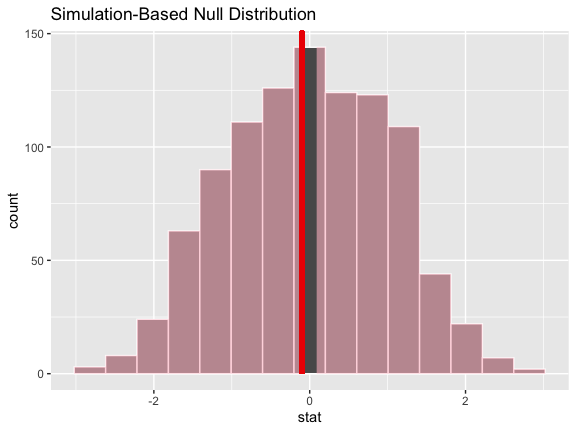Alternatively, visualizing the observed statistic using the theory-based null distribution,

``````visualize(null_dist_theory) +
shade_p_value(obs_stat = z_hat, direction = "two-sided")``````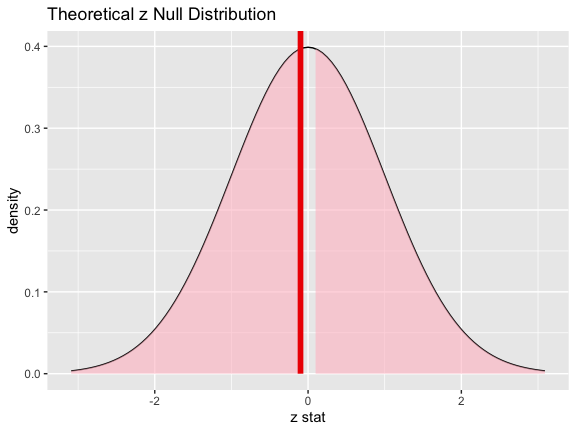Alternatively, visualizing the observed statistic using both of the null distributions,

``````visualize(null_dist, method = "both") +
shade_p_value(obs_stat = z_hat, direction = "two-sided")``````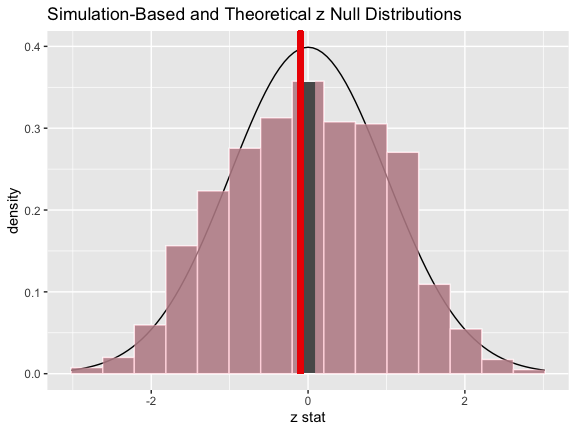Note that the above code makes use of the randomization-based null distribution.

Calculating the p-value from the null distribution and observed statistic,

``````null_dist %>%
get_p_value(obs_stat = z_hat, direction = "two-sided")``````
p_value
0.98

Note the similarities in this plot and the previous one.

The package also supplies a wrapper around `prop.test` to allow for tests of equality of proportions on tidy data.

``````prop_test(gss,
college ~ sex,
order = c("female", "male"))``````
statistic chisq_df p_value alternative lower_ci upper_ci
0 1 0.9964 two.sided -0.0918 0.0834

### One categorical (>2 level) - GoF

Calculating the observed statistic,

Note the need to add in the hypothesized values here to compute the observed statistic.

``````Chisq_hat <- gss %>%
specify(response = finrela) %>%
hypothesize(null = "point",
p = c("far below average" = 1/6,
"below average" = 1/6,
"average" = 1/6,
"above average" = 1/6,
"far above average" = 1/6,
"DK" = 1/6)) %>%
calculate(stat = "Chisq")``````

Alternatively, using the `observe()` wrapper to calculate the observed statistic,

``````Chisq_hat <- gss %>%
observe(response = finrela,
null = "point",
p = c("far below average" = 1/6,
"below average" = 1/6,
"average" = 1/6,
"above average" = 1/6,
"far above average" = 1/6,
"DK" = 1/6),
stat = "Chisq")``````

Then, generating the null distribution,

``````null_dist <- gss %>%
specify(response = finrela) %>%
hypothesize(null = "point",
p = c("far below average" = 1/6,
"below average" = 1/6,
"average" = 1/6,
"above average" = 1/6,
"far above average" = 1/6,
"DK" = 1/6)) %>%
generate(reps = 1000, type = "draw") %>%
calculate(stat = "Chisq")``````

Alternatively, finding the null distribution using theoretical methods using the `assume()` verb,

``````null_dist_theory <- gss %>%
specify(response = finrela) %>%
assume("Chisq")``````

Visualizing the observed statistic alongside the null distribution,

``````visualize(null_dist) +
shade_p_value(obs_stat = Chisq_hat, direction = "greater")``````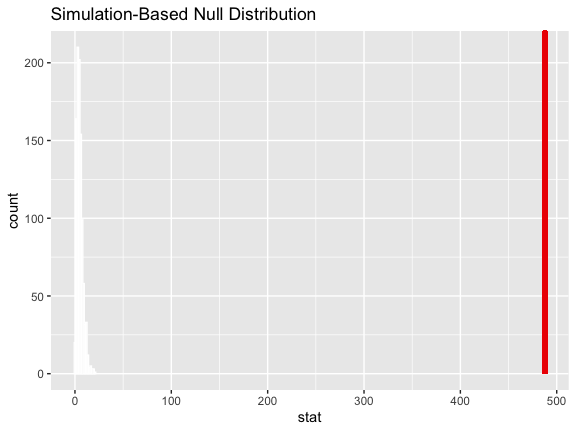Alternatively, visualizing the observed statistic using the theory-based null distribution,

``````visualize(null_dist_theory) +
shade_p_value(obs_stat = Chisq_hat, direction = "greater")``````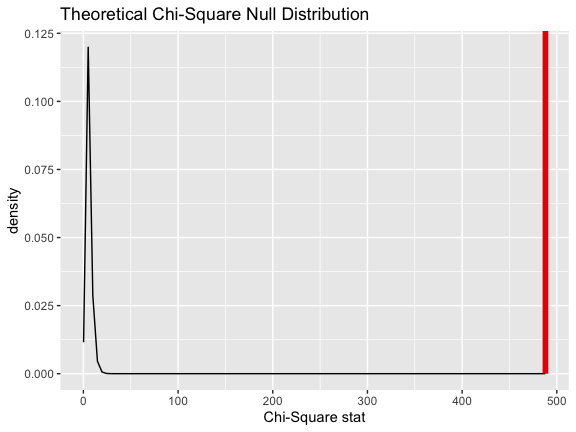Alternatively, visualizing the observed statistic using both of the null distributions,

``````visualize(null_dist_theory, method = "both") +
shade_p_value(obs_stat = Chisq_hat, direction = "greater")``````Note that the above code makes use of the randomization-based null distribution.

Calculating the p-value from the null distribution and observed statistic,

``````null_dist %>%
get_p_value(obs_stat = Chisq_hat, direction = "greater")``````
p_value
0

Alternatively, using the `chisq_test` wrapper:

``````chisq_test(gss,
response = finrela,
p = c("far below average" = 1/6,
"below average" = 1/6,
"average" = 1/6,
"above average" = 1/6,
"far above average" = 1/6,
"DK" = 1/6))``````
statistic chisq_df p_value
488 5 0

### Two categorical (>2 level): Chi-squared test of independence

Calculating the observed statistic,

``````Chisq_hat <- gss %>%
specify(formula = finrela ~ sex) %>%
hypothesize(null = "independence") %>%
calculate(stat = "Chisq")``````

Alternatively, using the `observe()` wrapper to calculate the observed statistic,

``````Chisq_hat <- gss %>%
observe(formula = finrela ~ sex, stat = "Chisq")``````

Then, generating the null distribution,

``````null_dist <- gss %>%
specify(finrela ~ sex) %>%
hypothesize(null = "independence") %>%
generate(reps = 1000, type = "permute") %>%
calculate(stat = "Chisq")``````

Alternatively, finding the null distribution using theoretical methods using the `assume()` verb,

``````null_dist_theory <- gss %>%
specify(finrela ~ sex) %>%
assume(distribution = "Chisq")``````

Visualizing the observed statistic alongside the null distribution,

``````visualize(null_dist) +
shade_p_value(obs_stat = Chisq_hat, direction = "greater")``````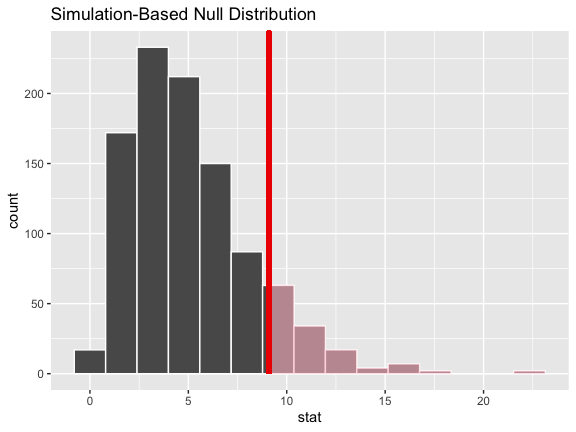Alternatively, visualizing the observed statistic using the theory-based null distribution,

``````visualize(null_dist_theory) +
shade_p_value(obs_stat = Chisq_hat, direction = "greater")``````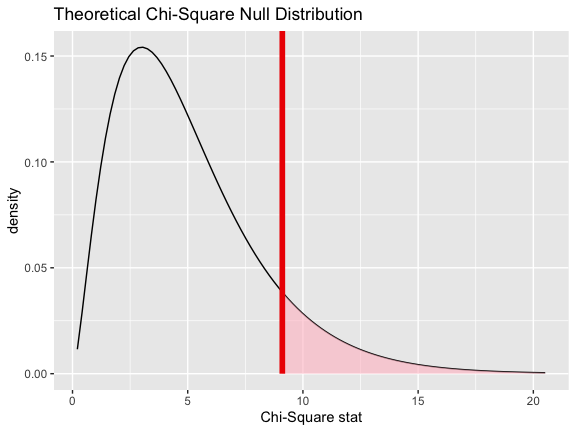Alternatively, visualizing the observed statistic using both of the null distributions,

``````visualize(null_dist, method = "both") +
shade_p_value(obs_stat = Chisq_hat, direction = "greater")``````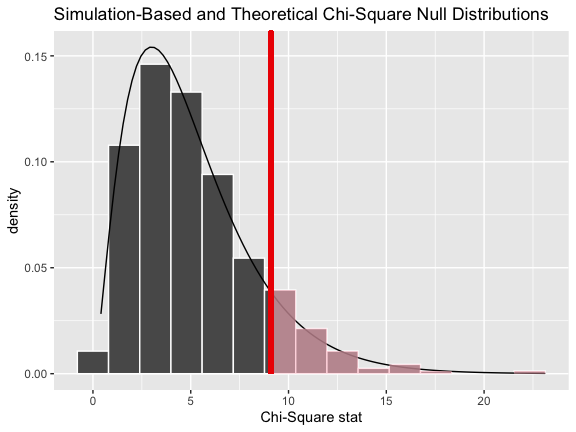Note that the above code makes use of the randomization-based null distribution.

Calculating the p-value from the null distribution and observed statistic,

``````null_dist %>%
get_p_value(obs_stat = Chisq_hat, direction = "greater")``````
p_value
0.118

Alternatively, using the wrapper to carry out the test,

``````gss %>%
chisq_test(formula = finrela ~ sex)``````
statistic chisq_df p_value
9.105 5 0.1049

### One numerical variable, one categorical (2 levels) (diff in means)

Calculating the observed statistic,

``````d_hat <- gss %>%
specify(age ~ college) %>%
calculate(stat = "diff in means", order = c("degree", "no degree"))``````

Alternatively, using the `observe()` wrapper to calculate the observed statistic,

``````d_hat <- gss %>%
observe(age ~ college,
stat = "diff in means", order = c("degree", "no degree"))``````

Then, generating the null distribution,

``````null_dist <- gss %>%
specify(age ~ college) %>%
hypothesize(null = "independence") %>%
generate(reps = 1000, type = "permute") %>%
calculate(stat = "diff in means", order = c("degree", "no degree"))``````

Visualizing the observed statistic alongside the null distribution,

``````visualize(null_dist) +
shade_p_value(obs_stat = d_hat, direction = "two-sided")``````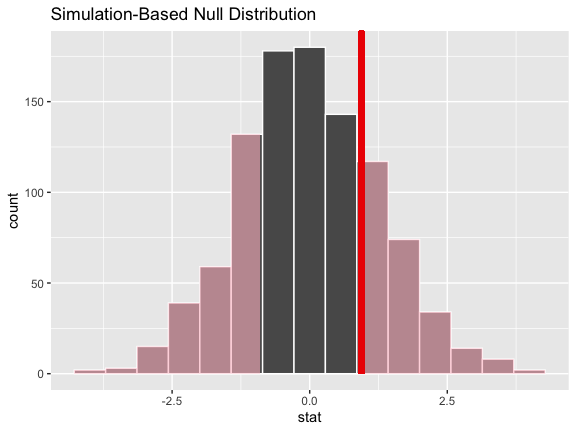Calculating the p-value from the null distribution and observed statistic,

``````null_dist %>%
get_p_value(obs_stat = d_hat, direction = "two-sided")``````
p_value
0.46

### One numerical variable, one categorical (2 levels) (t)

Finding the standardized observed statistic,

``````t_hat <- gss %>%
specify(age ~ college) %>%
hypothesize(null = "independence") %>%
calculate(stat = "t", order = c("degree", "no degree"))``````

Alternatively, using the `observe()` wrapper to calculate the observed statistic,

``````t_hat <- gss %>%
observe(age ~ college,
stat = "t", order = c("degree", "no degree"))``````

Then, generating the null distribution,

``````null_dist <- gss %>%
specify(age ~ college) %>%
hypothesize(null = "independence") %>%
generate(reps = 1000, type = "permute") %>%
calculate(stat = "t", order = c("degree", "no degree"))``````

Alternatively, finding the null distribution using theoretical methods using the `assume()` verb,

``````null_dist_theory <- gss %>%
specify(age ~ college) %>%
assume("t")``````

Visualizing the observed statistic alongside the null distribution,

``````visualize(null_dist) +
shade_p_value(obs_stat = t_hat, direction = "two-sided")``````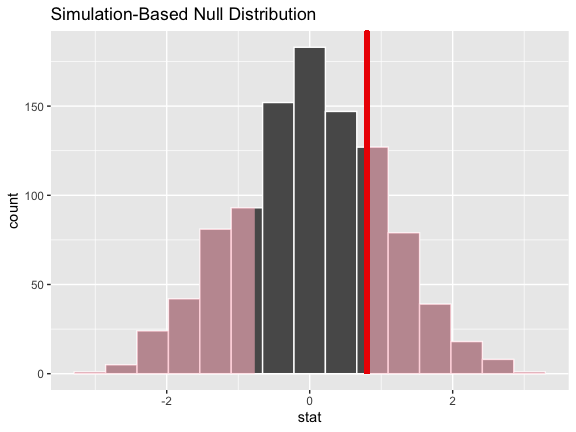Alternatively, visualizing the observed statistic using the theory-based null distribution,

``````visualize(null_dist_theory) +
shade_p_value(obs_stat = t_hat, direction = "two-sided")``````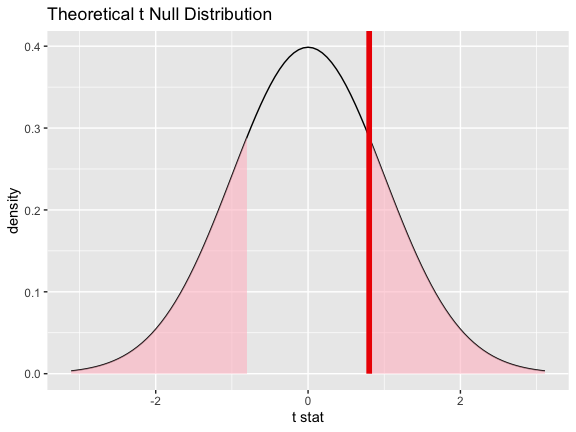Alternatively, visualizing the observed statistic using both of the null distributions,

``````visualize(null_dist, method = "both") +
shade_p_value(obs_stat = t_hat, direction = "two-sided")``````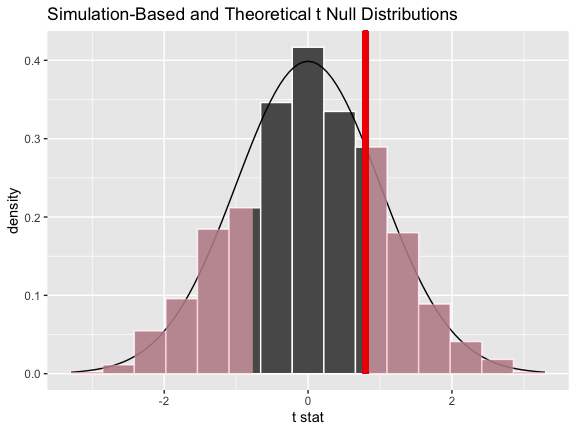Note that the above code makes use of the randomization-based null distribution.

Calculating the p-value from the null distribution and observed statistic,

``````null_dist %>%
get_p_value(obs_stat = t_hat, direction = "two-sided")``````
p_value
0.442

Note the similarities in this plot and the previous one.

### One numerical variable, one categorical (2 levels) (diff in medians)

Calculating the observed statistic,

``````d_hat <- gss %>%
specify(age ~ college) %>%
calculate(stat = "diff in medians", order = c("degree", "no degree"))``````

Alternatively, using the `observe()` wrapper to calculate the observed statistic,

``````d_hat <- gss %>%
observe(age ~ college,
stat = "diff in medians", order = c("degree", "no degree"))``````

Then, generating the null distribution,

``````null_dist <- gss %>%
specify(age ~ college) %>% # alt: response = age, explanatory = season
hypothesize(null = "independence") %>%
generate(reps = 1000, type = "permute") %>%
calculate(stat = "diff in medians", order = c("degree", "no degree"))``````

Visualizing the observed statistic alongside the null distribution,

``````visualize(null_dist) +
shade_p_value(obs_stat = d_hat, direction = "two-sided")``````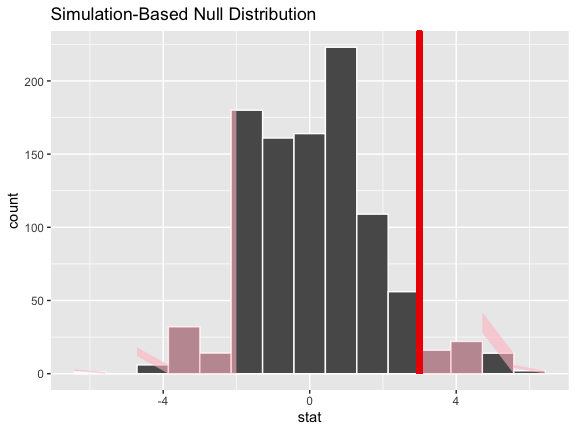Calculating the p-value from the null distribution and observed statistic,

``````null_dist %>%
get_p_value(obs_stat = d_hat, direction = "two-sided")``````
p_value
0.172

### One numerical, one categorical (>2 levels) - ANOVA

Calculating the observed statistic,

``````F_hat <- gss %>%
specify(age ~ partyid) %>%
calculate(stat = "F")``````

Alternatively, using the `observe()` wrapper to calculate the observed statistic,

``````F_hat <- gss %>%
observe(age ~ partyid, stat = "F")``````

Then, generating the null distribution,

``````null_dist <- gss %>%
specify(age ~ partyid) %>%
hypothesize(null = "independence") %>%
generate(reps = 1000, type = "permute") %>%
calculate(stat = "F")``````

Alternatively, finding the null distribution using theoretical methods using the `assume()` verb,

``````null_dist_theory <- gss %>%
specify(age ~ partyid) %>%
hypothesize(null = "independence") %>%
assume(distribution = "F")``````

Visualizing the observed statistic alongside the null distribution,

``````visualize(null_dist) +
shade_p_value(obs_stat = F_hat, direction = "greater")``````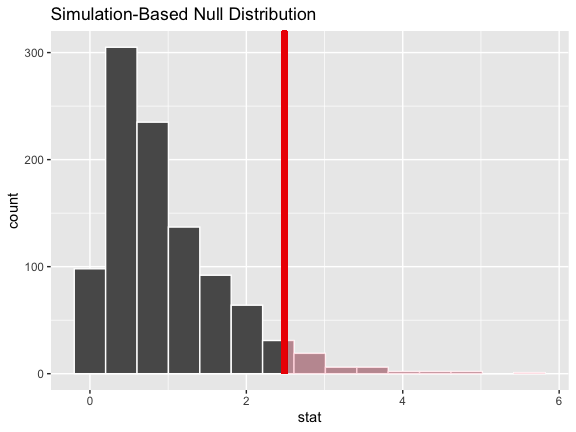Alternatively, visualizing the observed statistic using the theory-based null distribution,

``````visualize(null_dist_theory) +
shade_p_value(obs_stat = F_hat, direction = "greater")``````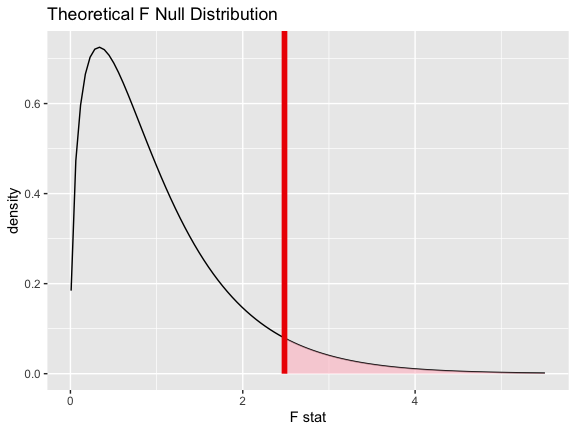Alternatively, visualizing the observed statistic using both of the null distributions,

``````visualize(null_dist, method = "both") +
shade_p_value(obs_stat = F_hat, direction = "greater")``````